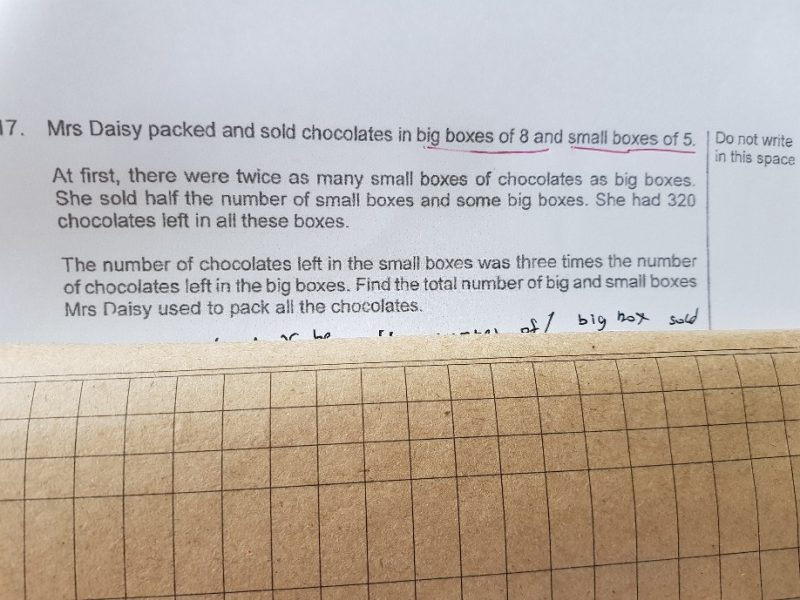QuestionEach big box contains 8 Chocolates

Each small box contains  6 chocolates

Small box = 2U

Big Box = 1U

After sale

Small box = 1U

Total chocolates left = 320

Small box contains 3C chocolates

Big box contains 1C chocolates

4C = 320

1C = 80

Chocolates in Big box = 80. Total big box left = 80/8 = 10.

Chocolates in Small box = 240. Total small box left = 240/5 = 48.

Initially

Small box = 2U = 2 x 48 = 96 boxes

Big Box    = 1U = 48 boxes

Total boxes = 48 + 96 = 144 boxes.

1 Reply 0 Likes

Dear Orckids,

Thank you.

0 Replies 0 Likes